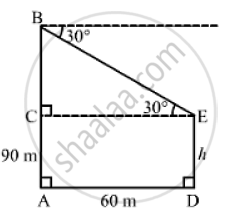# The Horizontal Distance Between Two Towers is 60 Meters. the Angle of Depression of the Top of the First Tower When Seen from the Top of the Second Tower is 30° Find the Height of the First Tower. - Mathematics

The horizontal distance between two towers is 60 meters. The angle of depression of the top of the first tower when seen from the top of the second tower is 30° . If the height of the second tower is 90 meters. Find the height of the first tower.

#### Solution

Let DE be the first tower and AB be the second tower.
Now, AB = 90 m and AD = 60 m such that CE = 60 m and ∠BEC = 30°.
Let DE = h m such that AC = h m and BC = (90- h)m.In the right ΔBCE,we have:

(BC)/(CE) = tan 30° = 1/ sqrt(3)

⇒ ((90-h )) /60 = 1/ sqrt(3)

⇒ (90-h ) sqrt(3) = 60

⇒ h sqrt(3) = 90 sqrt( 3 ) -60

⇒ h = 90- 60/ sqrt(3) = 90- 34.64 = 55.36 m

∴ Height of the first tower = DE = h = 55.36m

Concept: Heights and Distances
Is there an error in this question or solution?
Chapter 14: Height and Distance - Exercises

#### APPEARS IN

RS Aggarwal Secondary School Class 10 Maths
Chapter 14 Height and Distance
Exercises | Q 17

Share